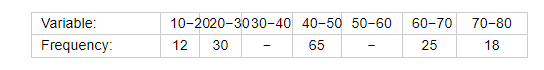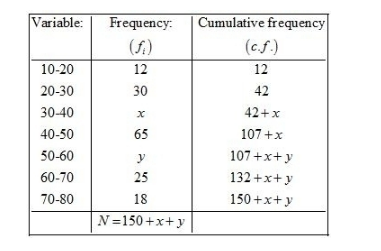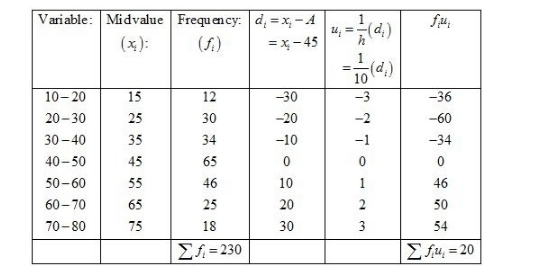# An incomplete distribution is given below :

Question:

An incomplete distribution is given below :You are given that the median value is 46 and the total number of items is 230.
(i) Using the median formula fill up missing frequencies.
(ii) Calculate the AM of the completed distribution.

Solution:

(i) Let the missing frequencies be and y.

First we prepare the following cummulative table.Here, $N=230$

So, $\frac{N}{2}=115$

It is given that the median is 46.

Therefore, $40-50$ is the median class.

$I=40, f=65, F=42+x$ and $h=10$

We know that

Median $=l+\left\{\frac{\frac{N}{2}-F}{f}\right\} \times h$

$46=40+\left\{\frac{115-(42+x)}{65}\right\} \times 10$

$6=\frac{(73-x) \times 10}{65}$

$39=(73-x)$

$x=73-39$

$x=34$

Also,

$N=230$

$150+x+y=230$

Putting the value of x, we get

$150+34+y=230$

$y=230-184$

$y=46$

Hence, the missing frequencies are 34 and 46.

(ii)

We may prepare the table as shown.We know that mean, $\bar{X}=A+h\left(\frac{1}{N} \sum f_{i} u_{i}\right)$

Now, we have $N=\sum f_{i}=230, \sum f_{i} u_{i}=20, h=10$ and $A=45$.

Putting the values in the above formula, we have

$\bar{X}=A+h\left(\frac{1}{N} \sum f_{i} u_{i}\right)$

$=45+10\left(\frac{1}{230} \times(20)\right)$

$=45+\frac{200}{230}$

$=45+0.87$

$=45.87$

Hence, the mean is 45.87.